# NCERT Solutions for Class 8 Maths Chapter 6 Squares and Square Roots

NCERT solutions for class 8 maths chapter 6 Squares and Square Roots - Mathematics is the combination of calculation, formulas & concepts. And the calculation is the backbone of mathematics. This chapter is based on the calculation with some concepts. The solutions of NCERT class 8 maths chapter 6 squares and square roots covers questions related to squares of numbers and square roots of numbers. The CBSE NCERT solutions for class 8 maths chapter 6 squares and square roots contains explanation to 4 exercises with 30 questions. Practicing questions is important to score good marks in mathematics and the solutions of NCERT helps for the same. The concepts studied in the chapter become more clear with the help of NCERT solutions for class 8 maths chapter 6 squares and square roots.  Square means a number will be multiplied 2-times by itself. For example:- If we want to calculate the square of 6, then the square will be 6×6 = 36,  likewise square of 5 = 5×5 = 25. The square root is just a reverse application of square, it means when a number multiplied 2-times by itself then it will result in the square and the root number of this result is called square root. For example- the square of 3 = 3×3 which is equal to 9 and similarly in the reverse manner square root of 9 is equal to 3. There is another way to understand this, Which number we should multiply 2 times by itself to gain 9, then the number which will strike in your mind will be 3.

.## Q.1 Find the perfect square numbers between

(i) and

(ii) and

(i) We know that

So clearly 36 is the perfect square number between 30 and 40.

(ii)  We know

So clearly it can be seen that there does not exist any perfect square number  between 50 and 60

## CBSE NCERT solutions for class 8 maths chapter 6 squares and square roots topic 6.2

We know that numbers which end with 0, 1, 4, 5, 6 or 9 at units place may be a perfect square number and all other number are not perfect square number.

Since the given number has 7 at units place hence this number is not a perfect square.

Q.1(ii) Can we say whether the following numbers are perfect squares? How do we know?

We have 23453.

Since this number ends with digit 3 so it cannot be a perfect square. (As we know a number must end with 0, 1, 4, 5, 6 or 9 for being a perfect square number.)

Q.1(iii) Can we say whether the following numbers are perfect squares? How do we know?

It is known that a number must end with 0, 1, 4, 5, 6 or 9 at units place for being a perfect square.

The given number ends with 8 so it is not a perfect square.

Q.1 (iv) Can we say whether the following numbers are perfect squares? How do we know?

Given number ends with digit 2.

We know that only a number ending with 0, 1, 4, 5, 6 or 9 at units place can be perfect square.

Therefore 222222 is not a perfect square number.

Q.1(v) Can we say whether the following numbers are perfect squares? How do we know?

Since the units place of a given number is 9, thus it may or may not be a perfect square number.

As we know a number ending with 0, 1, 4, 5, 6 or 9 at units place can be a perfect square number.

Q.1 (vi) Can we say whether the following numbers are perfect squares? How do we know?

It is known that the numbers that end with 0, 1, 4, 5, 6 or 9 at units place may be a perfect square.

Given number has 1 as the last digit so it may be a perfect square number.

Q.2 Write five numbers which you cannot decide just by looking at their units digit (or units place) whether they are square numbers or not.

The five numbers can be :- 521, 655, 124, 729, 1940 etc.

Basically, numbers ending with 0, 1, 4, 5, 6 or 9 at units place can be square numbers.

It is known that if a number has 1 or 9 in the units place, then it’s square ends in 1.

So squares of 161 and 109 would end with digit 1.

Q. Which of the following numbers would have digit 6 at unit place.

(i)

(ii)

(iii)

(iv)

(v)

We know that when a square number ends in 6, the number whose square will have either 4 or 6 in unit’s place.

So the required numbers are squares of  24, 26, 36, 34.

Q (i). What will be the “one’s digit” in the square of the following numbers?

1234

We have 1234.

Therefore one's digit is 6. (Since Square of digits ending with 4 gives 6 at units place.)

26387

We have 28367.

So the one's digit will be 9. (Since Square of digits ending with 7 gives 9 at units place.)

## Q (iii). What will be the “one’s digit” in the square of the following numbers?

We have 52698.

Its square will end with 4.        (Since square of a number ending with 8 ends with 4 at units place.)

We have 99880.

0  will be the “one’s digit” in the square of this number.  (Since the square of a number which ends with 0 will have 0 at units place.)

Q (v). What will be the “one’s digit” in the square of the following numbers?

4 will be the “one’s digit” in the square of the 21222.

Since the square of a number ending with 2 will give 4 at units place.

Q (vi) . What will be the “one’s digit” in the square of the following numbers?

6 will be the “one’s digit” in the square of 9106.

As we know that we get 6 at units place when we square a number ending with 6.

Q1.The square of which of the following numbers would be an odd number/an even number? Why?

(i) 727

(ii) 158

(iii) 269

(iv) 1980

We know that the squares of even numbers are even numbers and squares of odd numbers are odd numbers.

So squares of 727 and 269 will be odd numbers, and squares of 158 and 1980 will be even numbers.

Q2. What will be the number of zeros in the square of the following numbers?

(i) 60

(ii) 400

Square of a number having x number of zeros will have 2x number of zeros.

Thus,  (i) 60: Number of zeros will be 2.

(ii) 400: Number of zeros will be 4.

## Solutions of NCERT class 8 maths chapter 6 squares and square roots topic 6.3

In general, we can say that there are 2n non-perfect square numbers between the squares of the numbers n and (n + 1).

Thus between squares of 9 and 10, the number of natural numbers is 2(9) = 18

Similarly, between squares of 11 and 12, the number of natural numbers is 2(11) = 22

Q.2 How many non square numbers lie between the following pairs of numbers

(i)

(ii)

(iii)

In general, we can say that there are 2n nonperfect square numbers between the squares of the numbers n and (n + 1).

(i) The number of non-square numbers that lie between the square of 100 and 101 will be = 2(100)  = 200.

(ii) The number of non-square numbers that lie between the square of 90 and 91 will be = 2(90) = 180.

(iii) The number of non-square numbers that lie between the square of 1000 and 1001 will be = 2(1000) = 2000.

121

If it is a sum of successive odd natural numbers starting with 1, then it is a perfect square.

So we try to express 121 in successive integers. This can also be done by subtracting successive odd natural numbers from 121.

Applying the concept :-

121 - 1 = 120

120 - 3 = 117

117 - 5 = 112

112 - 7 = 105

105 - 9 = 96

96 - 11 = 85

85 - 13 = 72

72 - 15 = 57

57 - 17 = 40

40 - 19 = 21

21 - 21 = 0

Thus 121 is a perfect square.

Q(ii). Find whether each of the following numbers is a perfect square or not?

55

If it is a sum of successive odd natural numbers starting with 1, then it is a perfect square.

So we try to express 55 in successive integers. This can also be done by subtracting successive odd natural numbers from 55.

55 - 1 = 54  ;  54 - 3 = 51  ;  51 - 5 = 46  ;  46 - 7 = 39  ;  39 - 9 = 30  ;  30 - 11 = 19  ;  19 - 13 = 6.

Thus 55 is not a perfect square.

Q(iii). Find whether each of the following numbers is a perfect square or not?

81

If it is a sum of successive odd natural numbers starting with 1, then it is a perfect square.

So we try to express 81 in successive odd natural numbers. This can also be done by subtracting successive odd natural numbers from 81.

81 - 1 = 80

80 - 3 = 77

77 - 5 = 72

72 -  7 = 65

65 - 9 = 56

56 - 11 = 45

45 - 13 = 32

32 - 15 = 17

17 - 17 = 0

Thus 81 is a perfect square number.

## Q(iv). Find whether each of the following numbers is a perfect square or not?

49

If it is a sum of successive odd natural numbers starting with 1, then it is a perfect square.

So we try to express 49 in successive odd natural numbers. This can also be done by subtracting successive odd natural numbers from 49.

49 - 1 = 48

48 - 3 = 45

45 - 5 = 40

40 - 7 = 33

33 - 9 = 24

24 - 11 = 13

13 - 13 = 0.

Hence 49 is a perfect square number.

## Q (v). Find whether each of the following numbers is a perfect square or not?

69

If it is a sum of successive odd natural numbers starting with 1, then it is a perfect square.

So we try to express 69 in successive odd natural numbers. This can also be done by subtracting successive odd natural numbers from 69.

69 - 1 = 68

68 - 3 = 65

65 - 5 = 60

60 - 7 = 53

53 - 9 = 43

43 - 11 = 32

32 - 13 = 19

19 - 15 = 4

So the given number 69 is not a perfect square.

Q.1 Express the following as the sum of two consecutive integers.

(i)

(ii)

(iii)

(iv)

(i) 212 = 441 => 220 + 221

(ii) 132 = 169 => 84 + 85

(iii) 112 = 121 => 60 + 61

(iv) 192 = 361 => 180 + 181

No, the reverse is not true.

For e.g, the two consecutive number 20 and 21 gives a sum of 41. But we know that 41 is not a perfect square.

## NCERT solutions for class 8 maths chapter 6 squares and square roots topic 6.4

Q (i) . Find the squares of the following numbers containing 5 in unit’s place.

15

Assume a number with unit digit 5 = a5

=  = 10a(10a + 5) + 5(10a + 5) = 100a 2 + 50a + 50a + 25

= 100a(a + 1) + 25

= a(a + 1) hundred + 25

We will use this result here,

We have a5 = 15 , So a = 1

= 1(1+1)100 + 25 = 200 + 25 = 225

Q (ii). Find the squares of the following numbers containing 5 in unit’s place.

95

Assume a number with unit digit 5 = a5

=  = 10a(10a + 5) + 5(10a + 5) = 100a 2 + 50a + 50a + 25

= 100a(a + 1) + 25

= a(a + 1) hundred + 25

We are going to use this result here.

In this question a = 9

so,      = 9(9+1)hundred + 25

= 9000 + 25 = 9025

105

Assume a number with unit digit 5 = a5

=  = 10a(10a + 5) + 5(10a + 5) = 100a 2 + 50a + 50a + 25

= 100a(a + 1) + 25

= a(a + 1) hundred + 25.

We will use this concept here.

a = 10; so    = 10(10+1)hundred + 25

= 10(11)hundred + 25

= 11000 + 25 = 11025

Q (iv). Find the squares of the following numbers containing 5 in unit’s place.

205

Consider a number with unit digit 5, i.e.,    a5

=  = 10a(10a + 5) + 5(10a + 5) = 100a 2 + 50a + 50a + 25

= 100a(a + 1) + 25

= a(a + 1) hundred + 25.

Here a = 20

Hence     =    20(20+1)hundred + 25

=   20(21)hundred + 25 = 42000 + 25

=   42025

## is  ,a square root of ?

The detailed solution for the above-mentioned question is written here

Yes, Because after squaring -1 & 1 we get 1 in both the cases.

Since

is  a square root of

The solution for the above-written question is written here

Yes, because

is  a square root of

The solution for the above-written question is as follow

Yes, because

## By repeated subtraction of odd numbers starting from 1, find whether the following numbers are perfect squares or not? If the number is a perfect square then find its square root.

(i) 121

Consider . Then

81 - 1 = 80

80 - 3 = 77

77 -  5 = 72

72 - 7 = 65

65 - 9 = 56

56 - 11 = 45

45 - 13 = 32

32 - 15 = 17

17 - 17 = 0.

Since zero is obtained in the 9th step thus  = 9.

## By repeated subtraction of odd numbers starting from 1, find whether the following numbers are perfect squares or not? If the number is a perfect square then find its square root.

(ii) 55

We have .   Then

55 - 1 = 54

54 - 3 = 51

51 - 5 = 46

46 - 7 = 39

39 - 9 = 30

30 - 11 = 19

19 - 13 =  6.

Thus the given number is not a perfect square.

By repeated subtraction of odd numbers starting from 1, find whether the following numbers are perfect squares or not? If the number is a perfect square then find its square root.

(iii) 36

We have .  Then

36 - 1 = 35

35 - 3 = 32

32 - 5 = 27

27 - 7 = 20

20 - 9 = 11

11 - 11 = 0 .

We get zero on the 6th step so

## By repeated subtraction of odd numbers starting from 1, find whether the following numbers are perfect squares or not? If the number is a perfect square then find its square root.

(iv) 49

We have  . Then

49 - 1 =  48

48 - 3 = 45

45 - 5 = 40

40 - 7 = 33

33 - 9 = 24

24 - 11 = 13

13 - 13 = 0 .

We get zero on the 7th step so

By repeated subtraction of odd numbers starting from 1, find whether the following numbers are perfect squares or not? If the number is a perfect square then find its square root.

(v) 90

We have ,  then

90 - 1 = 89

89 - 3 = 86

86 - 5 =  81

81 - 7 = 74

74 - 9 = 65

65 - 11 = 54

54 - 13 = 41

41 - 15 = 26 ;

26 - 17 = 9.

So from the all above calculation, we can say that the given number is not a perfect square.

## Solutions of NCERT class 8 maths chapter 6 squares and square roots topic 6.5.4

Solution for the above-written question is as follows,

Yes.

The smallest 3-digit perfect square number = 100

which is the square of 10

the greatest 3-digit perfect square number is 961

which is the square of 31.

The smallest 4-digit square number is 1024

which is the square of 32

The greatest 4-digit number is 9801

which is the square of 99.

25600

The solution for the above-written question is as follows,

Since the given number has 5 digits. So the number of digits in square root :

100000000

The solution for the above-written question is as follows

Since the given number has a total of 9 digits.

Therefore  the number of digits in the square root will be :

36864

The solution for the above-written question is as follows

The given number has a total of 5 digits.

Thus the number of digits in the square root of this number

## CBSE NCERT solutions for class 8 maths chapter 6 squares and square root topic 6.7

Q (i). Estimate the value of the following to the nearest whole number.

A detailed explanation of the above-written question is as follows

We know that     and

So the whole number closest to  is 9.

Q (ii).Estimate the value of the following to the nearest whole number.

The detailed explanation of the above-written question is as follows

We know that       and   .

So the whole number closest to      is 32.

## Q (iii). Estimate the value of the following to the nearest whole number.

The detailed explanation for the above-written question,

We have  .

It is known that: and

So the closest whole number to      is 19.

Q. Estimate the value of the following to the nearest whole number.

(iv)

The detailed explanation for the above-written question is as follows

We have .

We know:                       and

So the closest whole number to        is 22.

## NCERT solutions for class 8 maths chapter 6 squares and square roots exercise 6.1

Q.1 What will be the unit digit of the squares of the following numbers?

(i) 81

(ii) 272

(iii) 799

(iv) 3853

(v) 1234

(vi) 26387

(vii) 52698

(viii) 99880

(ix) 12796

(x) 55555

The unit digit of the squares of the following numbers will be :-

(i) 81                                  :-    1

(ii) 272                               :-    4

(iii) 799                              :-    1

(iv) 3853                            :-    9

(v) 1234                             :-    6

(vi) 26387                          :-    9

(vii) 52698                         :-    4

(viii) 99880                        :-     0

(ix) 12796                         :-     6

(x) 55555                         :-      5

## 2. The following numbers are obviously not perfect squares. Give reason.

(i) 1057

(ii) 23453

(iii) 7928

(iv) 222222

(v) 64000

(vi) 89722

(vii) 222000

(viii) 505050

We know that only the numbers that end with 0, 1, 4, 5, 6 or 9 at units place can be perfectly square numbers.

Also, a perfectly square number has a number of zeros in multiple of 2.

Since these numbers have either odd no. of zeros or their unit place is 2, 3, 7, 8 thus they are not perfectly square numbers.

Q.3 The squares of which of the following would be odd numbers?

(i) 431

(ii) 2826

(iii) 7779

(iv) 82004

It is known that square of an odd number is always an odd number.

Therefore the square of 431 and 7779 will also be an odd number.

By observation, it is clear that the no. of zeros between 1 and 1 in LHS are equal to the no. of zeros between 1-2 and 2-1 in the RHS.

So,

and

Q.5 Observe the following pattern and supply the missing numbers.

The solution for the above-written question is as follows

By observation we get,

and

Q.6 Using the given pattern, find the missing numbers.

Patter is clearly visible.

First two numbers and the last two numbers are the consecutive numbers.

Moreover, the third number is obtained when the first is multiplied with the second number.

So required numbers can be found.

i.e., 4  5 = 20                and           6  7 = 42

hence

and

and

Q.7 Without adding, find the sum.

(i)

(ii)

(iii)

It is known that sum of odd cosecutive number starting from 1 is .

(i) n = 5       i.e.,

(ii) n = 10    i.e.,

(iii) n = 12    i.e.,

The solution for the above mentioned question is as follows:-

The splitted form of 49 (In increasing odd numbers) :- 1 + 3 + 5 + 7 + 9 + 11 + 13

8 (ii) Express 121 as the sum of 11 odd numbers.

The splitted form of number 121 (starting with odd numbers in increasing orders) = 1 + 3 + 5 + 7 + 9 + 11 + 13 +  15 + 17 + 19 + 21

Q. 9 (i)  How many numbers lie between squares of the following numbers?

We know that there are 2n non-perfect square numbers between the squares of the numbers n and (n + 1).

So for this question, n = 12

So total numbers that lie between squares of 12 and 13 are = 2(12) = 24.

It is known that there are 2n non-perfect square numbers between the squares of the numbers n and (n + 1).

So total number that lie between 25 and 26 will be = 2(25) = 50

## Q.9 (iii) How many numbers lie between squares of the following numbers?

We know that there are 2n non-perfect square numbers between the squares of the numbers n and (n + 1).

In this question, we have n = 99

Thus total number that lie between 99 and 100 = 2(99) = 198

CBSE NCERT solutions for class 8 maths chapter 6 squares and square roots exercise 6.2

Q.1 Find the square of the following numbers.

(i) 32

(ii) 35

(iii) 86

(iv) 93

(v) 71

(vi) 46

(i)  =  = 30(30 + 2) + 2(30 + 2)  =  30(32) + 2(32) = 960 + 64 = 1024

(ii)  =  = 30(30 + 5) + 5(30 + 5) = 30(35) + 5(35) = 1050 + 175 = 1225

(iii)  =  = 80(80 + 6) + 6(80 + 6) = 80(86) + 6(86) = 6880 + 516 = 7396

(iv)  =   = 90(90 + 3) + 3(90 +3) = 90(93) + 3(93) = 8370 + 279 = 8649

(v)   =    = 70(70 + 1) + 1(70 + 1) = 70(71) + 1(71)  =  4970 + 71 = 5041

(vi)    = = 40(40 + 6) + 6(40 + 6) = 40(46) + 6(46) = 1840 + 276 = 2110

Q.2(i). Write a Pythagorean triplet whose one member is.

6

For any natural number m > 1, 2m,  and  forms a Pythagorean triplet.

So if we take,

But value of m will not be an integer.

Now we take,

but the value of m will not be an integer.

If we take                       2m = 6

then                                 m = 3

Then               9 - 1 = 8           and            9 + 1  = 10.

Therefore the required triplet is 6, 8 and 10

Q.2 (ii) Write a Pythagorean triplet whose one member is.

14

For any natural number m > 1, 2m,  and  forms a Pythagorean triplet.

So if we take,

But then the value of m will not be an integer.

We take,

but the value of m will not be an integer.

If we take                       2m = 14

or                                     m = 7

Then               49 - 1 = 48           and            49 + 1  = 50.

Therefore the combination of number is 14, 48 and 50.

16

For any natural number m > 1, 2m,  and  forms a Pythagorean triplet.

So if we take,

But the value of m will not be an integer.

Now we take,

but the value of m will not be an integer.

If we take                       2m = 16

then                                 m = 8

Then               64 - 1 = 63           and            64 + 1  = 65.

Therefore the required numbers are 16, 63 and 65.

Q.2 (iv) Write a Pythagorean triplet whose one member is.

18

For any natural number m > 1, 2m,  and  forms a Pythagorean triplet.

So if we take,

But the value of m will not be an integer.

Now we take,

but the value of m will not be an integer.

If we take                       2m = 18

then                                 m = 9

Then               81 - 1 = 80           and            81 + 1  = 82.

Therefore the required combination is 18, 80 and 82

## Solutions of NCERT class 8 maths chapter 6 squares and square roots exercise 6.3

1 (i). What could be the possible ‘one’s’ digits of the square root of each of the following numbers?

9801

We know that square of digits ending with 1 and 9 gives 1 at units place.

So the number whose square ends in 1 = 1 & 9

So, possible unit digit of the square root of 9801 = 1 and 9.

1 (ii). What could be the possible ‘one’s’ digits of the square root of each of the following numbers?

99856

We know that square of digits ending with 4 and 6 gives 6 at its units place.

So possible ‘ones’ digits of the square root of  99856 are 4 and 6.

Q1 (iii). What could be the possible ‘one’s’ digits of the square root of each of the following numbers?

998001

We know that square of digits ending with 1 and 9 gives 1 at units place.

So the possible ‘one’s’ digits of the square root of 998001 are 1 and 9.

Q1 (iv). What could be the possible ‘one’s’ digits of the square root of each of the following numbers?

657666025

We know that square of a number ending with 5 gives 5 at its units place.

So the possible ‘one’s’ digits of the square root of 657666025 are 5.

Q.2 Without doing any calculation, find the numbers which are surely not perfect squares.

(i) 153

(ii) 257

(iii) 408

(iv) 441

As we know the units place of a perfect square cannot be 2, 3, 7, and 8.

So 153,  257,  408 are surely not perfect squares.

## Q.3  Find the square roots of and by the method of repeated subtraction.

(i)   For 100 :-  100 - 1 = 99

99 - 3 = 96

96 - 5 = 91

91 - 7 = 84

84 - 9 = 75

75 - 11 = 64

64 - 13 = 51

51 - 15 = 36

36 - 17 = 19

19 - 19 = 0.

We obtain zero at 10th step so

(ii)    For 169 :- 169 - 1 = 168

168 - 3 = 165

165 - 5 = 160

160 - 7 = 153

153 - 9 = 144

144 - 11 = 133

133 - 13 = 120

120 - 15 = 105

105 - 17 =  88

88 - 19 = 69

69 - 21 = 48

48 - 23 = 25;

25 - 25 = 0.

We obtain Zero at the 13th step so

Q.4 (i) Find the square roots of the following numbers by the Prime Factorisation Method.

(i) 729

By prime factorisation, we know that

or

Thus the square root of 729 is 27.

## Q.4 (ii) Find the square roots of the following numbers by the Prime Factorisation Method.

400

By prime factorization, we get

or

Thus the square root of 400 is 20

Q4 (iii). Find the square roots of the following numbers by the Prime Factorisation Method.

1764

We have 1764, by prime factorization we get

or

Thus the square root of 1764 is 42.

Q.4 (iv) Find the square roots of the following numbers by the Prime Factorisation Method.

4096

We have 4096, by prime factorization:

or          .

So the square root of 4096 is 64.

Q.4 (v) Find the square roots of the following numbers by the Prime Factorisation Method.

(v) 7744

We have in 7744. By prime factorization, we get

or

Thus the square root of 7744 is 44.

Q.4 (vi)  Find the square roots of the following numbers by the Prime Factorisation Method.

(vi) 9604

We have in 9604. By prime factorization we get,

or

Hence the square root of 9604 is 98.

5929

The solution for the above-written question is as follows

Prime factorization of number 5929,

or           .

Thus, the square root of 5929 is 77.

## Q4 (viii). Find the square roots of the following numbers by the Prime Factorisation Method.

(viii) 9216

The solution for the above-written question is as follows

prime factorization of 9216,

or           .

Thus, the square root of 9216 is 96.

Q.4  Find the square roots of the following numbers by the Prime Factorisation Method.

(ix) 529

The solution for the above-written question is as follows

We have 529.

Prime factorization gives

So square root of 529 is 23.

Q.4 Find the square roots of the following numbers by the Prime Factorisation Method.

(x) 8100

The solution for the above-written question is as follows

We have in 8100.

By prime factorization, we get :

or                                                     .

So square root of 8100 is 90.

Q.5  For each of the following numbers, find the smallest whole number by which it should be multiplied so as to get a perfect square number. Also find the square root of the square number so obtained

(i) 252

(ii) 180

(iii) 1008

(iv) 2028

(v) 1458

(vi) 768

(i) 252 :    Prime factorisation of 252 = .

To make pairs we will multiply 252 with 7.

So the number is 1764 and its square root is 42.

(ii) 180 :     Prime factorisation of 180 = .

To make it perfect square, multiply by 5.

So the number is 900 and its square root is 30.

(iii) 1008 :   Prime factorization of 1008 gives = .

To make pairs we need to multiply it by 7.

So the number we get is 7056 and its square root is 84.

(iv) 2028 :    Prime factorisation of 2028 = .

To make pairs we multiply the number by 3.

So the number obtained is 6084 and its square root is 78.

(v)  1458 :   Prime factorisation of 1458 gives =

To make pairs we need to multiply the number by 2.

So the number obtained is 2916 and its square root is 54.

(vi) 768  :     Prime factorisation of 768 gives =

To make pairs we need to multiply the given number by 6.

So the required number is 4608 and its square root is 48.

Q.6 For each of the following numbers, find the smallest whole number by which it should be divided so as to get a perfect square. Also find the square root of the square number so obtained.

(i) 252

(ii) 2925

(iii) 396

(iv) 2645

(v) 2800

(vi) 1620

(i) 252: Prime factorization of 252 gives = .

For making pairs we will divide the given number by 7.

The obtained number is 36 and its square root is 6.

(ii) 2925: Prime factorization of 2925 gives =

To make pairs divide the given number by 13.

So the obtained number is 225 and its square root is 15.

(iii) 396: Prime factorization if 396 =

For obtaining perfect square number we need to divide the given number by 11.

So the required number is 36 and its square root is 6.

(iv) 2645: Prime factorization of 2645 =

We need to divide the given number by 5 to obtain the perfect square number.

So the obtained number is 529 and its square root is 23.

(v) 2800:      Prime factorization of 2800  =

To make pairs we need to divide 2800 by 7.

So the required number is 400 and its square root is 20.

(vi) 1620: Prime factorization of 1620 gives =

To make pairs divide the given number by 5.

We get , number = 324 and its square root = 18.

## Q.7  The students of Class VIII of a school donated  in all, for Prime Minister’s National Relief Fund. Each student donated as many rupees as the number of students in the class. Find the number of students in the class.

Let the number of students in a class be x.

According to question,

Number of student = money donated by each of the students

So total money donated =

or

Prime factorization of

So the number of students in the class = 49.

Q.8  2025 plants are to be planted in a garden in such a way that each row contains as many plants as the number of rows. Find the number of rows and the number of plants in each row.

The total number of plants = No. of rows  No. of plants in 1 row.

Since in this case  no.of rows = no. of plants in each row.

Thus let us assume the number of rows to be x.

Then the equation becomes   :

Prime factorization of 2025 gives =

So value of x is = 45.

Hence no. of rows = 45; and no. of plants in each row = 45.

## Q.9 Find the smallest square number that is divisible by each of the numbers   and .

This has to be done in two steps. First, we will find LCM of given numbers, then we will make it a perfect square.

So the LCM of 4, 9, 10 is   180.                                  4 = 22  ;           9 = 33   ;             10 =  25

Prime factorisation of 180 gives = .

To make it a perfect square we need to multiply it with 5.

So, the smallest square number which is divisible by each of the numbers 4, 9 and 10 = 900.

Q.10 Find the smallest square number that is divisible by each of the numbers and .

This has to be done in two steps. First we will find LCM of given numbers, then we will make it perfect square.

So the LCM of 8, 15, 20 is 120   .                                  8 = 222  ;           15 = 35   ;             20 =  225

Prime factorisation of 120 gives =  .

To make it a perfect square we need to multiply it with 30.

So the smallest square number that is divisible by each of the numbers 4, 9 and 10 is 3600.

## NCERT solutions for class 8 maths chapter 6 squares and square roots exercise 6.4

Q.1 (i) Find the square root of each of the following numbers by Division method.

2304

The detailed explanation for the above-written question is as follows,

We will find the square root using the division method.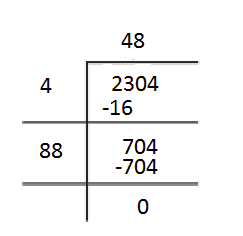## Squares and Square Roots Excercise: 6.4

### Question:

Q.1 (ii) Find the square root of each of the following numbers by Division method.

4489

The square root of 4489 is 67.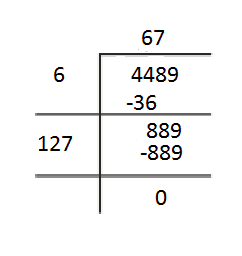## Q.1 (iii) Find the square root of each of the following numbers by Division method.

3481

The square root of 3481 is obtained as 59.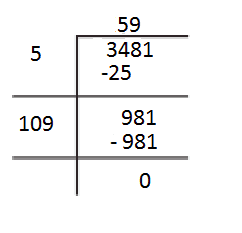## Q1 (iv). Find the square root of each of the following numbers by Division method.

529

The detailed solution for the above-written question is as follows

The square root of 529 is 23.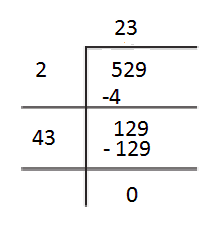Q1 (v).Find the square root of each of the following numbers by Division method.

3249

The detailed solution for the above-written question is as follows,

The square root of 3249 is 57.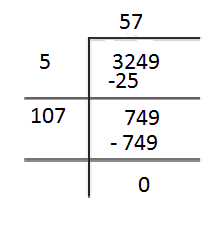Q1 (vi). Find the square root of each of the following numbers by Division method.

1369

The detailed solution for the above-written question is as follows,

The square root of 1369 is 37.

## Q1 (vii). Find the square root of each of the following numbers by Division method.

5776

The solution for the above-written question is as follows,

The square root of 5776 is 76.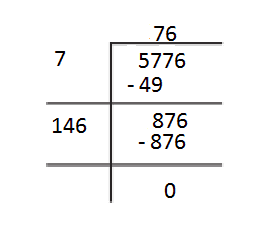## Q1 (viii).Find the square root of each of the following numbers by Division method.

7921

The detailed solution for the above-written question is as follows,

The square root of 7921 is 89.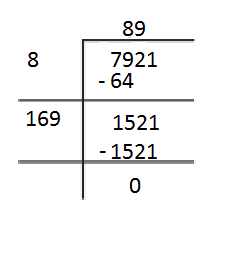## Q1 (ix).Find the square root of each of the following numbers by Division method.

576

The detailed solution for the above-written question is as follows,

The square root of 576 is 24.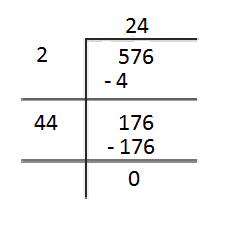## Q1 (x). Find the square root of each of the following numbers by Division method.

1024

The detailed solution for the above-written question is as follows,

The square root of 1024 is 32.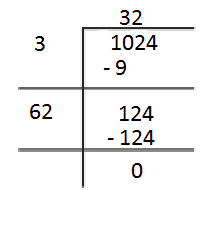Q.1(xi) Find the square root of each of the following numbers by Division method.

3136

The detailed solution for the above-written question is as follows,

The square root of 3136 is 56.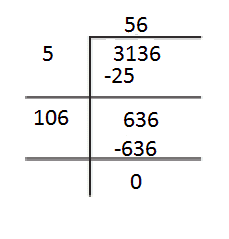Q.1 (xii) Find the square root of each of the following numbers by Division method.

900

The detailed solution for the above-written question is as follows

The square root of 900 is 30.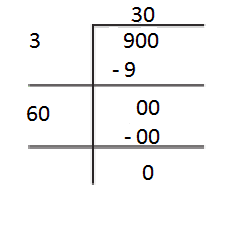Q.2 Find the number of digits in the square root of each of the following numbers (without any calculation).

(i) 64

(ii) 144

(iii) 4489

(iv) 27225

(v) 390625

(i) 64:-     The number of digits in the square root will be

(ii) 144:-   The number of digits in the square root will be

(iii) 4489:- The number of digits in the square root will be

(iv) 27225:-  The number of digits in the square root will be

(v) 390625:-   The number of digits in the square root will be

Q.3. Find the square root of the following decimal numbers.

(i)

(ii)

(iii)

(iv)

(v)

The detailed solution for the given questions as follows

(i) Square root of 2.56 using division method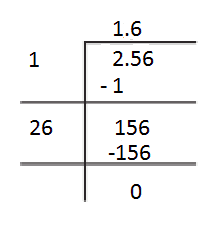(ii)  The square root of 7.29 using division method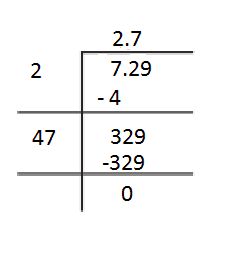(iii) The square root of 51.84 using division method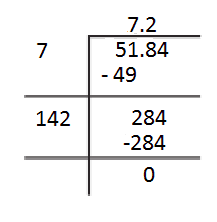(iv)  The square root of 42.25 using division method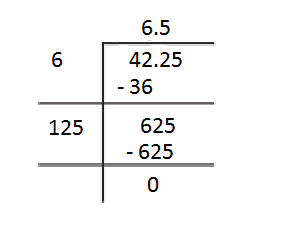(v)  The square root of 31.36 using division method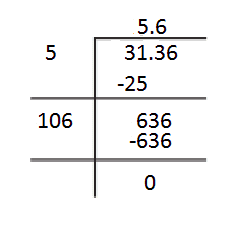4. Find the least number which must be subtracted from each of the following numbers so as to get a perfect square. Also find the square root of the perfect square so obtained.

(i) 402

(ii) 1989

(iii) 3250

(iv) 825

(v) 4000

(i)    402 :-     It can be seen that 2 is remainder. So we will subtract 2 from 402.

The required number is 400 and its square root is 20.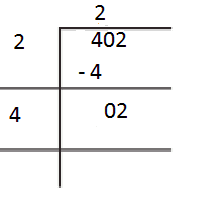(ii) 1989:- It can be seen that 53 is remainder here. So we will subtract 53 from 1989 in order to make it a perfect square.

The required number is 1936 and its square root is 44.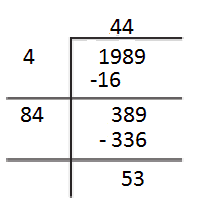(iii) 3250 :- It can be seen that 1 is remainder. So we will subtract 1 from 3250.

The required number is 3249 and its square root is 57.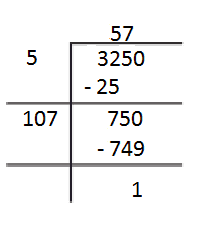(iv)  825:-  It can be seen that 41 is remainder. So we will subtract 41 from 825 to make it a perfect square number.

The required number is 784 and its square root is 28.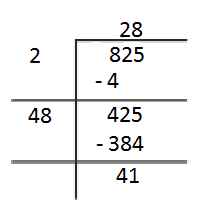(v) 4000 :- It can be seen that 31 is remainder here. So we will subtract 31 from 4000.

The required number is 3969 and its square root is 63.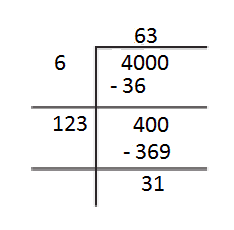Q.5 Find the least number which must be added to each of the following numbers so as to get a perfect square. Also find the square root of the perfect square so obtained.

(i) 525

(ii) 1750

(iii) 252

(iv) 1825

(v) 6412

(i) 525:-    It is clearly visible that if we add 4 to the given number, the remainder will become zero.

So obtained number is 529 and its square root is 23.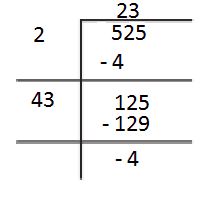(ii) 1750:-    It is clearly visible that if we add 14 to the given number, the remainder will become zero.

So the obtained number is 1764 and its square root is 42.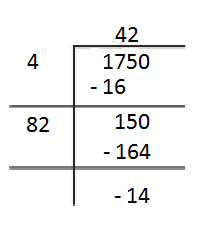(iii) 252:-  It is clearly visible that if we add 4 to the given number, the remainder will become zero.

So the obtained number is 256 and its square root is 16.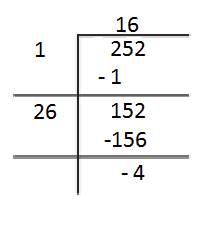(iv) 1825:-  It is clearly visible that if we add 24 to the given number, the remainder will become zero.

So the obtained number is 1849 and its square root is 43.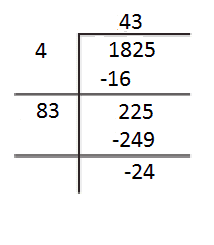(v)  6412:-   It is clearly visible that if we add 149 to the given number, the remainder will become zero.

So the obtained number is 6561 and its square root is 81.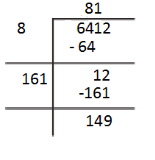Let the length of the side of a square be x m.

Area of square =

So equation becomes :

By prime factorisation of 441.

441 =

Thus x = 21.

So the length of the side of square = 21 m.

7 (a). In a right triangle

(a)  If , , find

Using Pythagoras theorem,

By prime factorisation of 100 :-

We get,                                   AC = 10cm

Q.7 (b) In a right triangle

If  find

Using Pythagoras theorem,

or

or

or

Prime factorisation of 144 gives :-

Hence,                        AB = 12 cm

Q.8  A gardener has 1000 plants. He wants to plant these in such a way that the number of rows and the number of columns remain same. Find the minimum number of plants he needs more for this.

It is given that the number of rows and the number of columns are the same.

Let a number of rows or number of columns be x.

The number of plants required  =

The gardener has 1000 plants.

We need to find a perfect square just greater than 1000.

We know,                      and

So the minimum plants needed by gardener = 1024 - 1000 = 24 plants.

Q.9  There are 500 children in a school. For a P.T. drill they have to stand in such a manner that the number of rows is equal to number of columns. How many children would be left out in this arrangement?

Given that the number of rows is equal to the number of columns.    i.e., in the form of

So the number of students that can stand in this order will be the perfect square number just less than 500.

We know that                    and

So the number of students that would be left out in this arrangement = 500 - 484 = 16 students.

## NCERT solutions for class 8 maths: Chapter-wise

 Chapter -1 NCERT solutions for class 8 maths chapter 1 Rational Numbers Chapter -2 Solutions of NCERT for class 8 maths chapter 2 Linear Equations in One Variable Chapter-3 CBSE NCERT solutions for class 8 maths chapter 3 Understanding Quadrilaterals Chapter-4 NCERT solutions for class 8 maths chapter 4 Practical Geometry Chapter-5 Solutions of NCERT for class 8 maths chapter 5 Data Handling Chapter-6 CBSE NCERT solutions for class 8 maths chapter 6 Squares and Square Roots Chapter-7 NCERT solutions for class 8 maths chapter 7 Cubes and Cube Roots Chapter-8 Solutions of NCERT for class 8 maths chapter 8 Comparing Quantities Chapter-9 NCERT solutions for class 8 maths chapter 9 Algebraic Expressions and Identities Chapter-10 CBSE NCERT solutions for class 8 maths chapter 10 Visualizing Solid Shapes Chapter-11 NCERT solutions for class 8 maths chapter 11 Mensuration Chapter-12 NCERT Solutions for class 8 maths chapter 12 Exponents and Powers Chapter-13 CBSE NCERT solutions for class 8 maths chapter 13 Direct and Inverse Proportions Chapter-14 NCERT solutions for class 8 maths chapter 14 Factorization Chapter-15 Solutions of NCERT for class 8 maths chapter 15 Introduction to Graphs Chapter-16 CBSE NCERT solutions for class 8 maths chapter 16 Playing with Numbers

## Important points of chapter squares and square roots-

The questions discussed in the NCERT solutions for class 8 maths chapter 6 squares and square roots are based on the following points

• If is expressed as , where 'm' and 'n' are natural numbers, then m is a square number.
• All square numbers end with 0, 1, 4, 5, 6 or 9 at unit’s place.
• Square numbers can only have even number of zeros at the end.
• The square root is just a reverse application of square.
• There are two integral square roots of a perfect square number.